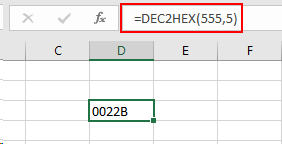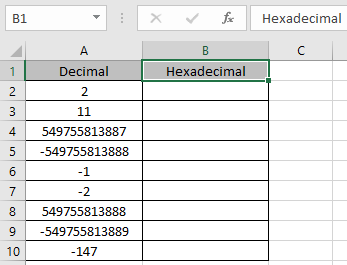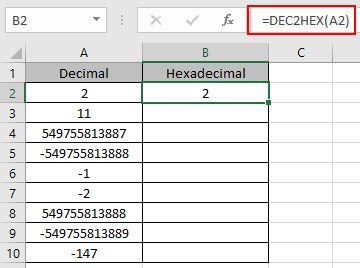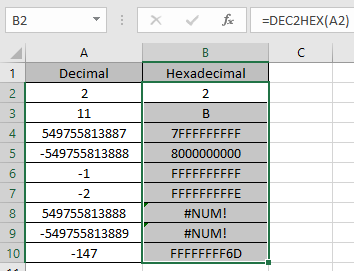# How to use the DEC2HEX Function in Excel

DEC2HEX function in excel is used to convert decimal representation of numbers of radix 10 to hexadecimal numbers (radix = 16).

The table shown below shows you some of the most used base & their radix of Alpha - numeric characters

 Base radix Alpha-Numeric Characters decimal 2 0 - 1 octal 8 0 - 7 Decimal 10 0 - 9 hexadecimal 16 0 - 9 & A - F hexatridecimal 36 0 - 9 & A - Z

Hexadecimal uses the decimal numbers and six extra symbols

Decimal number is representation of a number of radix 10. 10 digits are used in representation of a decimal number 0 - 9. Where as hexadecimal number representation uses the 10 decimal numbers 0, 1, 2, 3, 4, 5, 6, 7, 8 & 9 and six extra symbols A (= 10 in decimal), B, C, D, E & F. The below table will help you understand better

 Decimal Hexadecimal 0 0 1 1 2 2 3 3 4 4 5 5 6 6 7 7 8 8 9 9 10 A 11 B 12 C 13 D 14 E 15 F

You can see from the above table that decimal value 10 converted to hexadecimal comes out to be A.

The DEC2HEX function converts the decimal number of radix 10 to the hexadecimal number of radix 16.
Syntax:

=DEC2HEX ( number, [Places] )

Number : decimal number which is to be converted to hexadecimal
[Places] : [optional] number where result expressed upto the number.

The input decimal number to the function should be between - 536870912 to + 536870911. The resulting hexadecimal number will have upto 10 characters (10 bits), where first number express the sign of the number (positive or negative) & Other 9 express the value after the sign. ( 1 for negative & 0 for positive)To get the hexadecimal number, [places] number must have have sufficient places to express the hexadecimal number or else it returns the #NUM! Error. The decimal negative number is processed using two's complement notation and ignore [places] number if any.

Now let’s get more understanding of the function via using them in some examples.Here we have some decimal values in hexadecimal Column. We need to convert these decimal numbers to hexadecimal value.

Use the formula in Decimal column:

=DEC2HEX (A2)

A2 : number provided to the function as cell reference Values to the DEC2HEX function is provided as cell reference.The hexadecimal representation of 2 of base 10 (decimal) is 2 of base 16 (hexadecimal).
Now copy the formula to other cells using the Ctrl + D shortcut key.As you can see here the DEC2HEX function returns the results of the input values.

Notes:

1. Numbers can be given as argument to the function directly without quotes or cell reference in excel.
2. The function doesn’t consider the [places] number in case of a negative decimal number.
3. The number must be a valid decimal number between - 536870912 to + 536879011.
4. If the input [places] number is not an integer, it is truncated by the function.
5. The function returns the hexadecimal value for the max value of  7FFFFFFFFF & minimum value upto - 8000000000.
6. The function returns the #NUM! Error
1. If the input decimal number is less than - 549,755,813,888 or greater than + 549,755,813,887.
2. If the input number [places] is zero or negative.
3. If the input number [places] is not sufficient for the resulting positive hexadecimal number.
7. The function returns the #VALUE! Error
1. If the input number is text or non-numeric.
2. If the input [places] number is text or non-numeric.

Hope you understood how to use DEC2HEX function and referring cell in Excel. Explore more articles on Excel mathematical conversion functions here. Please feel free to state your query or feedback for the above article.

Related Articles:

How to use the DEC2BIN Function in Excel

How to use the BIN2OCT Function in Excel

How to use the BIN2HEX Function in Excel

How to use the DEC2OCT Function in Excel

How to use the DEC2HEX Function in Excel

How to use the OCT2BIN Function in Excel

How to use the OCT2DEC Function in Excel

How to use the OCT2HEX Function in Excel

How to use the HEX2BIN Function in Excel

How to use the HEX2OCT Function in Excel

How to use the HEX2DEC Function in Excel

Popular Articles:

If with wildcards

Vlookup by date

Join first and last name in excel

Count cells which match either A or B

Terms and Conditions of use

The applications/code on this site are distributed as is and without warranties or liability. In no event shall the owner of the copyrights, or the authors of the applications/code be liable for any loss of profit, any problems or any damage resulting from the use or evaluation of the applications/code.